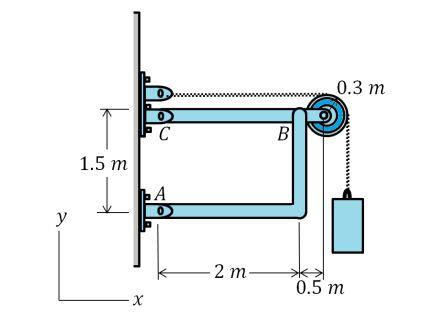# Consider the frame shown in figure The suspended cylinder has a mass of 80 kg. 1) Determine the x...

## Question:

Consider the frame shown in figure The suspended cylinder has a mass of 80 kg.

1) Determine the x and y components of reaction at pin B on BC using scalar notation.

2) Determine the x and y components of force at pin C using scalar notation.## Reaction Force

The force whose direction is given in counter of load operating on the body is termed as a reaction force. The reaction force in a body is given by relating the equilibrium condition of forces acting on the system.

Become a Study.com member to unlock this answer! Create your account

Given data

• Radius of pulley is: {eq}r = 0.3\;{\rm{m}} {/eq}
• Mass of cylinder is: {eq}m = 80\;{\rm{kg}} {/eq}
• Distance from point A to C is:...Action and Reaction Forces: Law & Examples

from

Chapter 4 / Lesson 21
227K

Action force is force acting in one direction. Reaction force is force acting in the opposite direction. Learn more about Newton's Third Law as it explains action and reaction forces through several examples, and test your knowledge with quiz questions.